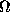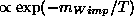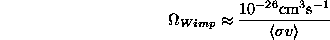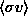The Net Advance of Physics: The Nature of Dark Matter, by Kim Griest -- Section 4.

Next: Non-thermal Relics Up: Brief Survey Previous: Brief Survey

# Thermal Relics as Dark Matter (Wimps)

Among the particle dark matter candidates an important

distinction is whether the particles were created thermally in the

Early Universe, or whether they were created non-thermally in a

phase transition. Thermal and non-thermal relics have a different

relationship between their relic abundanceand their properties

such as mass and couplings, so the distinction is especially important

for dark matter detection efforts. For example, the Wimp class of

particles can be defined as those particles which are created

thermally, while dark matter axions come mostly from

non-thermal processes.

In thermal creation one imagines that early on, when the Universe

was at very high temperature, thermal equilibrium obtained, and

the number density of Wimps (or any other particle species) was

roughly equal to the number density of photons. As the Universe

cooled the number of Wimps and photons would decrease together

as long as the temperature remained higher than the Wimp mass.

When the temperature finally dropped below the Wimp mass,

creation of Wimps would require being on the tail of the thermal

distribution, so in equilibrium, the number density of Wimps would

drop exponentially. If equilibrium were

maintained until today there would be very few Wimps left, but at

some point the Wimp density would drop low enough that the

probability of one Wimp finding another to annihilate would

become small. (Remember we must assume that an individual

Wimp is stable if it is to become the dark matter.) The Wimp

number density would ``freeze-out" at this point and we would be

left with a substantial number of Wimps today. Detailed evolution

of the Boltzmann equation can be done for an accurate prediction

[Section 6.2], but roughlywhereis the thermally averaged cross section for two Wimps to

annihilate into ordinary particles. The remarkable fact is that for, as required by the dark matter problem, the annihilation

cross sectionfor any thermally created particle turns out to be

just what would be predicted for particles with electroweak scale

interactions. Thus the ``W" in ``Wimp". There are several

theoretical problems with the Standard Model of particle physics

which are solved by new electroweak scale physics such as

supersymmetry. Thus these theoretical problems may be clues that

the dark matter does indeed consist of Wimps. Said another way,

any stable particle which annihilates with an electroweak scale

cross section is bound to contribute to the dark matter of the

Universe. It is interesting that theories such as supersymmetry,

invented for entirely different reasons, typically predict just such a

particle.

The fact that thermally created dark matter has weak scale

interactions also means that it may be within reach of accelerators

such as LEP at CERN, and CDF at Fermilab. After all, these

accelerators were built precisely to probe the electroweak scale.

Thus many accelerator searches for exotic particles are also

searches for the dark matter of the Universe. Also, due to the weak

scale interactions, Wimp-nuclear interaction rates are within

reach of many direct and indirect detection methods (see Section

6).Next: Non-thermal Relics Up: Brief Survey Previous: Brief Survey